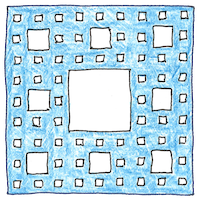# 1975 Fractal

## Fractal

A fractal is a geometric figure that is similar to itself at all scales. Bending a line into a fractal shape in two dimensions approaches a surface. Breaking a surface into a fractal shape in three dimensions approaches a solid. Benoit Mandelbrot coined the term fractal from the Latin word meaning “fractured.”

## Sierpinski carpet

Divide a square into nine subsquares, remove the center square, and repeat indefinitely. The result is a perimeter containing zero area. Its interior, eventually, is totally empty.

## Koch snowflake

Replace the middle third of each side of an equilateral triangle with two sides of an equilateral triangle pointing outward. Repeating indefinitely results in a finite area bounded by an infinitely long line.

## Mandelbrot set

The Mandelbrot set contains the complex numbers that when added to z2 continue to give finite values as z goes from zero to infinity, so they can be mapped to a two-dimensional colored image. Mandelbrot did not invent the Mandelbrot set. It was invented by Adrien Douady who also invented the Douady rabbit, which is similar to the Mandelbrot set but has a pair of floppy ears instead of buds, and each ear has a pair of ears, ad infinitum.

## Natural fractals

A human brain, a head of cauliflower, balls of crumpled paper, the inner surface of human lungs, distribution of galaxy clusters, the coast of Ireland, approximate fractals of different fuzzinesses, which is why fractals are used in computer graphic systems to approximate natural objects.

Benoit Mandelbrot created the first theory of roughness and proposed fractals as models of roughness in the real world.

The result of writing, producing draft after draft, also resembles a fractal; however, its depth is limited by the writer’s time and patience.